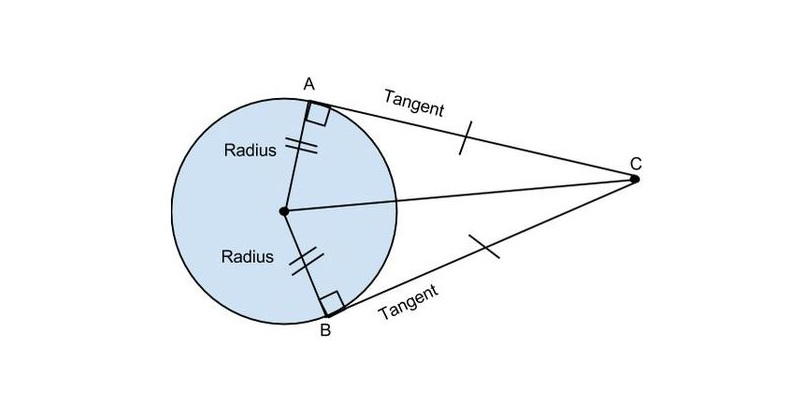# Quiz: How Much You Know About Circle And Tangents?

Questions : 12 | Total Attempts: 388 | Recent Updated: 06-Aug-2020A circle is a set of those points in a plane that are at a given constant from a given fixed point in the plane. The fixed point is called the center of the circle and the constant distance of every point on the circle from its center is called the radius of the circle. It is a two-dimensional closed figure. So take this quiz and know some interesting theoretical questions about Circle and Tangents.

### Questions Excerpt

1. Which of the following is the longest chord of the circle?

B. Diameter

C. Secant

D. Tangent

2. A line that intersects a circle in two distinct points is called ......

A. Chord

B. Tangent

C. Secant

D. Sector

3. A tangent to a circle is a line that intersects the circle at exactly......

A. Three points

B. Two Points

C. Zero points

D. One point

4. ........ is the distance once around the circle.

B. Circumference

C. Diameter

D. Sector

5. A line segment that goes from one point to another on the circle's circumference is called a ......

A. Chord

B. Arc

C. Secant

D. Tangent

6. A part of the circumference is called .....

A. Chord

B. Arc

D. Sector

7. Two tangents can always be drawn to a circle from any point outside the circle, and these tangents are ..... in length.

A. Different

B. Similar

C. Equal

D. None of these

8. Two or more circles that have the same center, but different radii is called .....

A. Tangent circle

B. Concentric circle

C. Congruent circle

D. All of these

9. Congruent circles are those circles that have the same ...... but different .....

B. Centers , Circumference

C. Circumference, Chord

10. How many parts are in a circle?

A. 5

B. 7

C. 8

D. 10

11. Who found the first theorems relating to circles around 650 BC?

A. Thales

B. Ahmes

C. Both of these

D. None of these

12. What is the formula for the area of the circle?

A. 2π r

B. π r²

C. 3/4 π r²

D. 1/2π r²

## Accounting Basics Quiz and Test

Numbers are the only constant in our lives and are an essential part of our lives! No matter what we do, or where we are, you will see these numbers are the same. Even in our daily activities, numbers are more useful than we can imagine. Let us talk

## Orthographic Projection Quiz: How Much Do You Know Orthographic Projection?

Geometry, is undoubtedly a very interesting and captivating subject, as we all can see and must have studied. When it comes to science, we can all agree that there is no shortage of unexpected factors. We all studied these two concepts together in sc

## Decimals Quiz for 6th Grade Student

A decimal is a fragment that has been written in a particular way. Instead of writing 1/2, you could use the decimal 0.5 to symbolize the fraction, with the 0 withinside the one's vicinity and the 5 withinside the tenth's vicinity. Decimal derives fr

## Place Arrangement Trivia Quiz Questions and Answers

Place arrangement generally refers to the positioning of a person or object in a manner indicated by a set of information given. One has to understand the order of placement and then attempt questions by following the given information. It is a part

## Analogy Or Relationship Test Trivia Quiz Questions and Answers

In analogy tests, the relationship between two given words is established and applied to the other words. The type of relationship may vary, so while attempting such questions the first step is to identify the type of relationship, which can be any o

## Ultimate Trivia Quiz on Angle

In plane geometry, two rays that share a common endpoint and formed a figure is called angle and the two rays are called the sides of the angles. The endpoint of the rays called the vertex of the angle. The symbol angle is ∠. In general, angles are m

## Similarity Of Triangle Trivia Quiz

Triangle is a two-dimensional figure and basic shape of the geometry. Two triangles are said to be similar if their corresponding angles are equal and their corresponding sides are in the same ratio. Two line segments are divided proportionally when

## Quiz: How Much You Know About Triangle?

Triangle is the closed basic shape in the geometry which has three edges and three vertices. There are three types of the triangle which are classified on the basis of the length of their sides and it is also classified on the basis of the internal d